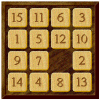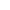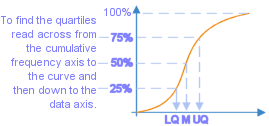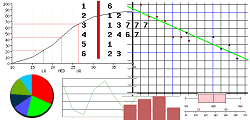Go to content## Summary/BackgroundYou must be clear about how to find the median, upper quartile (UQ) and lower quartile (LQ) from a cumulative frequency graph. To find the median you should start from the point halfway up to the total frequency on the vertical axis, draw a line across to the curve and then down to the horizontal axis. To find the LQ and UQ you start from a quarter and from three-quarters the way up on the vertical axis.
Remember that the Interquartile range = Upper quartile - Lower quartile.You should know how to draw a number of other statistical diagrams too, including:

scatter diagrams, pie charts, bar charts, line graphs, stem & leaf diagrams and box & whisker plots

## Software/Applets used on this pageThis page uses jsMath
You can get a better display of the maths by downloading special TeX fonts from jsMath. In the meantime, we will do the best we can with the fonts you have, but it may not be pretty and some equations may not be rendered correctly.

## Glossary

### axis

One of two straight lines on a graph from which measurements are taken. One axis (the y axis) is vertical; the other (the x axis) is horizontal.

### frequency

Of a function: the rate of repetition of a periodic function.
In statistics: the number of occurances of a value

### graph

A diagram showing a relationship between two variables.
The diagram shows a vertical y axis and a horizontal x axis.

### interquartile range

A measure of dispersion; the difference between the upper and lower quartiles; Q3-Q1.

### median

the middle value of a set of data that has been arranged in order of magnitude; half the data values are above it and half are below.

### quartile

In an ordered set of data 25% are below the first (Q1), 50% are below the second (Q2), 75% are below the third (Q3).

### range

In Statistics: the difference between the largest and smallest values in a data set; a simple measure of spread or variation
In Pure Maths: the values that y can take given an equation y=f(x) and a domain for x.

### union

The union of two sets A and B is the set containing all the elements of A and B.

Full Glossary List

## This question appears in the following syllabi:

SyllabusModuleSectionTopicExam Year
AQA AS Maths 2017StatisticsData Presentation and InterpretationCumulative Frequency-
AQA AS/A2 Maths 2017StatisticsData Presentation and InterpretationCumulative Frequency-
AQA GCSE (9-1) Higher (UK)S: StatisticsS3: Grouped Data, Histograms and Cumulative FrequencyCumulative Frequency-
CBSE X (India)Statistics and ProbabilityStatisticsCumulative frequency graph-
CIE IGCSE (9-1) Maths (0626 UK)9 StatisticsE9.7 Cumulative Frequency and Box-PlotsCumulative Frequency-
Edexcel AS Maths 2017StatisticsData Presentation and InterpretationCumulative Frequency-
Edexcel AS/A2 Maths 2017StatisticsData Presentation and InterpretationCumulative Frequency-
Edexcel GCSE (9-1) Higher (UK)S: StatisticsS3: Grouped Data, Histograms and Cumulative FrequencyCumulative Frequency-
GCSE Higher (UK)StatisticsDisplaying dataCumulative frequency-
OCR AS Maths 2017StatisticsWorking with DataCumulative Frequency-
OCR GCSE (9-1) Higher (UK)12: Statistics12.02b: Grouped DataCumulative Frequency-
OCR MEI AS Maths 2017StatisticsWorking with DataCumulative Frequency-
Universal (all site questions)DDisplaying dataCumulative frequency-